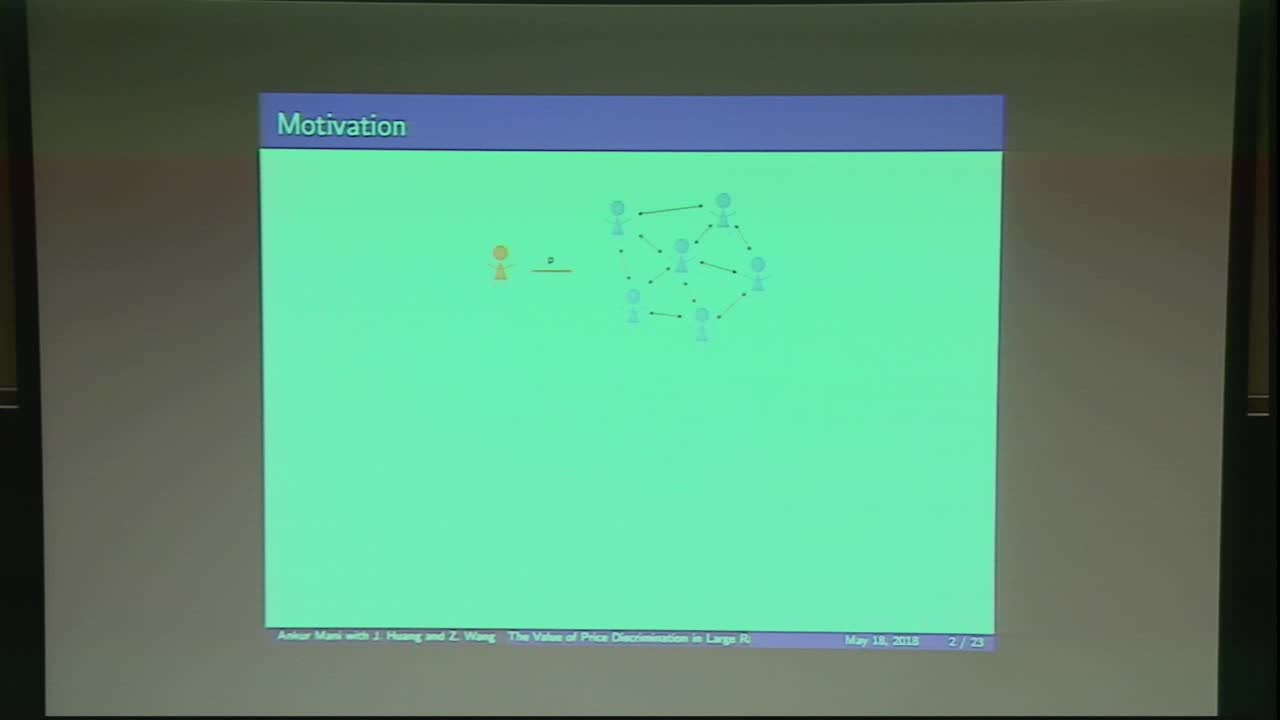## The Value of Price Discrimination in Large Random Networks

May 18, 2018
IMA
Presenters: Ankur Mani

Watch Video

#### Abstract

In this paper, we focus on the issue of price discrimination based upon position of individuals in the social network. The problem of price discrimination based upon position in the social network has received significant attention in recent years. However, the question of interest in the research has been to characterize and identify optimal prices and profits in deterministic networks and the complexity of computing optimal prices. We quantify the value of price discrimination as the difference in a monopolist's profit under two prices: (i) optimal discriminative prices, and (ii) optimal uniform prices.

We study the value of such price discrimination in large random graphs, in particular Erdos-Renyi graphs. To model the scenario, we consider a sequence of games played among consumers and monopolist selling divisible goods with positive network externalities. In each game the consumers choose their consumption level based upon the price and the consumption of their peers in the social network. The size of the consumer population is increasing in the index of sequence and the consumer social networks are Erdos-Renyi graphs. We identify tight upper and lower bounds on asymptotic profit rates and the value of price discrimination in the size of the network and show that these rates demonstrate phase transitions as the density of the network changes. Our results show that:
1. the value of price discrimination is sub linear in the size of the network for all network densities suggesting that the added profits from price discrimination is not a positive fraction of the total profit.
2. the value of price discrimination is the highest when the average degree of the network is independent of the size of the network. In this case, the network has a giant component with sufficient imbalance that a few stars influence many in the giant component. In this case price discrimination may be valuable for decently large networks. However, such networks are very sparse as compared to real world social networks.
3. if the average degree decreases with the size of the network then the network is very fragmented and so the influence of all nodes is limited within their components, leading to small gains from price discrimination.
4. if the average degree increases with the size of the network (at least as the logarithm of the network size) then the network tends to be very balanced and all positions have roughly equal influence. Therefore, the value of price discrimination decreases and is asymptotically zero.